Reach Us+44-1522-440391
Cubic Spline Regression of J-shaped Dose-Response Curves with Likelihood-based Assignments of Grouped Exposure Levels | OMICS International
Journal of Biometrics & Biostatistics

Like us on:

All submissions of the EM system will be redirected to Online Manuscript Submission System. Authors are requested to submit articles directly to Online Manuscript Submission System of respective journal.

# Cubic Spline Regression of J-shaped Dose-Response Curves with Likelihood-based Assignments of Grouped Exposure Levels

Kunihiko Takahashi1*, Hiroyuki Nakao2 and Satoshi Hattori3

1Department of Biostatistics, Nagoya University Graduate School of Medicine, Japan

2Center for Public Health Informatics, National Institute of Public Health, Japan

3Biostatistics Center, Kurume University, Japan

*Corresponding Author:
Kunihiko Takahashi
Department of Biostatistics
Nagoya University Graduate School of Medicine
65 Tsurumai-cho, Showa-ku, Nagoya, 466-8550, Japan
Tel: +81-52-744-2489
Fax: +81-52-744-2488
E-mail: [email protected]

Received Date: November 18, 2013; Accepted Date: November 28, 2013; Published Date: November 30, 2013

Citation: Takahashi K, Nakao H, Hattori S (2013) Cubic Spline Regression of J-shaped Dose-Response Curves with Likelihood-based Assignments of Grouped Exposure Levels. J Biomet Biostat 4:181. doi: 10.4172/2155-6180.1000181

Copyright: © 2013 Takahashi K, et al. This is an open-access article distributed under the terms of the Creative Commons Attribution License, which permits unrestricted use, distribution, and reproduction in any medium, provided the original author and source are credited.

Visit for more related articles at Journal of Biometrics & Biostatistics

#### Abstract

In epidemiological studies that measure the risk at different levels of exposure, the data is often only available for the analyses that summarized the response data in grouped exposure intervals. In typical methods, the midpoints are used as the assigned exposure levels for each interval. Results of the analysis with grouped data may be sensitive to the assignment of the exposure levels. In this paper, we propose a procedure for assessing J-shaped associations based on the likelihood-based assignment of values to grouped intervals of exposure, and applying the cubic spline regression models. Numerical illustrations and comparisons based on simulations showed that the proposed procedure can yield better estimates for curves than those obtained using the typical assignment method based on the midpoints of each interval.

#### Keywords

Dose-response; Spline regression model; Grouped exposure interval; Dose assignment; Likelihood; J-shaped curve

#### Introduction

In epidemiological studies, it is often necessary to determine the relationship between exposure levels and the risk of disease. However, the data on exposure levels are often available in intervals because they are generally not recorded for each individual subject. For example in studies on the association between alcohol consumption and the risk of disease, researchers often treat the exposure levels as intervals when they interview participants about their consumption levels, but they are unable to obtain the exact values as continuous variables. Also in traditional meta-analysis based on aggregated data, it is not possible to obtain the original data, and the published articles do not include enough data. In such situations, meta-analysis of observational studies often has to rely on the summarized data where the exposure levels are grouped into intervals available from research reports.

Table 1 summarizes data from a study of the association between alcohol consumption and all-cause mortality, which was conducted by Lin et al. . The alcohol intake of current drinkers was classified into five groups: non-drinkers, alcohol intake of 0.1-22.9 g/day, 23.0- 45.9 g/day, 46.0-68.9 g/day, and ≥ 69.0 g/day. Table 2 summarizes the characteristics of two studies of coffee consumption and stroke, by Bidel et al.  and Grobbee et al. . They were included into a metaanalysis of 11 studies by Larsson and Orsini , where the categories used for coffee consumption differed among the studies. Moreover the reference category was assigned to non-drinkers in Grobbee et al. , whereas 0-2 cups/day were used in Bidel et al. , and hence the meanings of the reported relative risks (RRs) were different and it was inappropriate to combine them directly.

Table 1: Relative risks of death from all causes as well as alcohol intake among men in the JACC study in Japan (for details, see Lin et al. ).

Table 2: Characteristics of two studies of coffee consumption and stroke that were included in a meta-analysis .

Studies that measure the risk at different levels of exposure are usually analyzed based on a trend estimate by linear (or loglinear) regression analysis. When performing a regression analysis of summarized response data that are grouped into intervals, many researchers use the pre-assigned exposure levels from historical data or the midpoint values of each interval 5[]. Results of regression analysis with grouped data may be sensitive to the assignment of the exposure levels. Recently, Takahashi and Tango  proposed a method for assigning values by applying the likelihood approach, and they showed the procedure can produce a more accurate linear regression coefficient than the typical procedure which uses the midpoint values.

On the other hand, some studies have reported that the risk of disease has a nonlinear relationship with the exposure level. For example, it is known that the association between alcohol and coronary heart disease  or total mortality  may be depicted as a J-shaped curve. Some evidence of a J-shaped association has also been reported recently between coffee consumption and the risk of stroke . Curve-fitting methods based on a fractional polynomial model or a spline model has often been applied to these nonlinear dose-response associations for regression [9-11]. Di Catelnuovo et al.  applied fractional polynomials to fit the association between alcohol intake and the RR of total mortality in a meta-analysis of 34 prospective studies. On the other hand, for example, Larsson and Orsini  performed a dose-response meta-analysis and detected a potentially nonlinear association between coffee consumption and stroke using a cubic spline model, and cubic spline regression models may have many advantages over polynomials .

In this paper, we propose a procedure for assessing nonlinear associations between exposure levels and the risk of disease from a summarized grouped data, which is based on the assignment of levels to grouped exposure intervals by applying the likelihoodbased assignment procedure proposed in Takahashi and Tango . In particular, we focus on the restricted cubic spline model that was described in Orsini et al.  and Larsson and Orsini  for J-shaped dose-response curves. We demonstrate how to estimate a J-shaped curve from the grouped summarized data using only four or five class intervals. Also this procedure can provide the log relative risk on each exposure levels relative to that of 0, even if the level of reference category for reported RRs was not 0. We provide some numerical illustrations and comparisons based on simulations with typical assignments to determine the effects of exposure level assignments.

#### Methods

Likelihood based assignment of levels for grouped exposure intervals

In some cohort studies with N individuals, the data on exposure xi of each individual i is summarized in a table of grouped exposure interval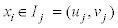and the corresponding summarized response data with the frequency Nj, the number of cases aj, the log value of the relative risk yj and its standard error sj for each interval Ij, j=0,1,…,m (Table 3), where the lower endpoint u0 is known but the upper endpoint vm is unknown. Nj is given as the number of individuals, or the person-time, and yj is sometimes shown as the adjusted values of some covariates. sj can also be estimated from the confidence interval of the risks if the standard error of the logRR is not reported . In case-control studies, however, the number of controls bj is also given and yj is the log value of the odds ratio (OR).

In typical methods, the midpoints are used as the assigned exposure levels for each interval Ij, (j=1,2,…,m) (here after midpoints assignment). On the other hand, we assign the exposure level based on the summarized data according to the procedure proposed by Takahashi and Tango  as follows. We assume that the exposure levels of all individuals in the study are a set of random variables (x1, x2,…, xN) and that a power transformation of the exposure,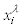, is obtained from a common normal distribution N(μ,σ2) with the mean μ and variance σ2, where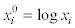for λ=0. Given this assumption, the frequency Nj provides a log-likelihood based on a binomial distribution for the distribution of x=xi. The unknown parameters λ, μ, σ2 and vm can be estimated by maximizing the log-likelihood. Based on the estimated distribution of x=xi with the probability density function f(x) such as xλ ~ N(μ,σ2) , the assigned exposure level dj for the jth interval Ij=(uj,vj) is calculated as the mean of its truncated distribution: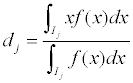(j=0,1,…,m) (hereafter likelihood-based assignment).

Nonlinear association modeling using cubic splines

Splines are smooth functions that can assume virtually any shape, and the most useful type of spline is generally a cubic spline function, which is restricted to be smooth at the junction of each cubic polynomial . In epidemiological studies, a restricted cubic spline model has been often applied to nonlinear dose-response data. As noted by Larsson and Orsini , a restricted cubic spline with three knots was recently applied to a potential nonlinear association that was depicted as a J-shaped curve. However, in some studies, such as that in Table 2, the exposure level of the reference group is not assigned on x=0. Thus, in this paper, we consider a cubic spline model for the log relative risk on the exposure x, logRR(x), that satisfies logRR(d0)=0 using assigned exposure levels d0 for the reference interval I0, as follows: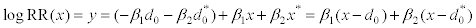(1)

where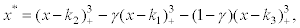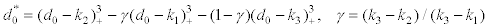with fixed knots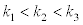and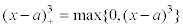First, we construct an approximate covariance estimate for the adjusted log relative risks from a fitted table that conforms to the values proposed by Greenland and Longnecker , and we construct a variance-covariance matrix Σ. In this step, we assume the assigned exposure levels dj and standard errors sj for each interval Ij to be fixed. The coefficients b of the restricted cubic spline model (1) are estimated using generalized least-squares regression with Σ, i.e.,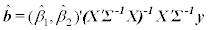and the estimated variance-covariance matrix of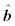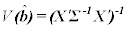where A’ and A-1 imply the transpose and inverse matrices of A, and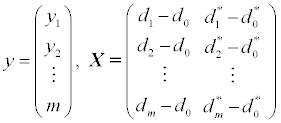respectively. Note that, if we need to estimate a spline model for the log relative risk on x relative to x=0, logRR0(x), when the reported y implies that relative to the reference x=d0, we can determine the spline model as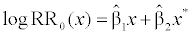(2)

A crucial problem in spline regression is knot placement . One simple approach is to have the observations xi determine the positions of the knots . Some studies such as those by Larsson and Orsini , Harrell et al.  and Orsini et al. , placed knots at fixed percentiles in the data. Therefore, we examine a procedure here for selecting the positions of three knots among the assigned exposure levels dj (j=1,2,…,m) with the exception of d0 of the reference group I0. Thus, we assign the knots k1=d1, k2=d2, k3=d3 when m=3. If m>3, we require a procedure that is based on likelihood. Under the normal assumption of generalized linear regression, y~Nm (Xb,Σ), we can derive the loglikelihood function of b and evaluate the likelihood of each resulting model for several candidate knot positions. Thus, the putatively better knots k1, k2, and k3 are placed in a position that maximizes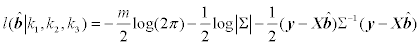(3)

Application

First for the data in Table 1 with person-years for Nj, the likelihoodbased assignment procedure assigned the exposure levels as dj=0, 14.26, 34.20, 56.09, and 86.41 g/day with the estimated parameters λ=0.5 and vm=139.90. When using the number of individuals for Nj, they are assigned as dj=0, 14.27, 34.21, 56.09, and 86.41 g/day with the estimated parameters λ=0.5 and vm=140.00. Thus, they differed from the midpoints assignment of dj=0, 11.5, 34.45, 57.45, and 82.8 (as 1.2 times the lower boundary for the open-ended upper category) or 80.45 (as assuming the open-ended upper category has the same amplitude as the adjacent category) g/day for each Ij. By using the exposure levels of dj=0, 14.26, 34.20, 56.09, and 86.41 g/day, likelihood procedure (3) selected k1=14.26, k2=34.20, and k3=86.41 as the knots, and the coefficients were estimated as β1=-4.88×10-3 and β2=-5.46×10-6, respectively, while the midpoints assignment with d4=80.45 estimated the coefficients as β1=-2.51×10-3 and β2=-1.36×10-5 with k1=11.5, k2=57.45 and k3=80.45 (Figure 1).

Figure 1: Fitted curve for the log of the adjusted relative risk logRR0 of allcause mortality associated with alcohol intake, as reported by Lin et al.  in Table 1 (black line: the likelihood-based assignment; gray line: the midpoints assignment). Dashed lines represent the 95% confidence intervals based on the asymptotic normal theory.

Next for the data in Table 2, the exposure levels were assigned by the likelihood-based assignment as dj=1.65, 3.56, 5.44, and 7.94 cups/ day with λ=2/3 and vm=14.4 in Bidel et al.  where the intervals were assumed as 0 ≤ x<2.5, 2.5 ≤ x<4.5, 4.5 ≤ x<6.5, and 6.5 ≤ x, respectively. k1=3.56, k2=5.44, and k3=7.94 were set as the knots, the coefficients were estimated as β1=-0.131 and β1=-0.024, respectively, and we can determine the estimated curve of logRR0 from the model (2) (Figure 2). On the other hand, the midpoints assignment with d0=0.0 estimated β1=-0.085 and β1=-0.023.

Figure 2: Cubic spline curves logRR0(x)=-0.2x - 0.05x* for the true model with knots (k1, k2, k3) of (I) (3, 5, 7) and (II) (2.3, 3.2, 10.5)).

Simulation

In this section, we discuss the simulation studies conducted to assess our proposed procedure, wherein we used cubic spline regression for the nonlinear association with the likelihood-based assignments in grouped exposure intervals. Two cubic spline curves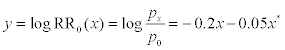(4)

with three knots (k1, k2, k3)=(I) (3, 5, 7) and (II) (2.3, 3.2, 10.5) were assumed to be the true model for the association between exposure x and the log relative risk y in a cohort study, respectively, where px and p0 are the probabilities of being a case with the exposure x and x=0, respectively (Figure 3). We considered interval for grouping, 0 ≤ x<2, 2 ≤ x<4, 4 ≤ x<6, and x ≥ 6, such as that the number of knots in each interval Ij was at most one for curve I, whereas the interval 2 ≤ x<4 had two knots, i.e., k1=2.3 and k2=3.2, for curve II.

Figure 3: Cubic spline curves logRR0(x)=-0.2x - 0.05x* for the true model with knots (k1, k2, k3) of (I) (3, 5, 7) and (II) (2.3, 3.2, 10.5)).

We set the population size N=2,000 and the probability p0=0.05 for the reference x=0. We generated a set of exposures x={x1, x2,…,x2,000} from a truncated normal distribution of N(3.5, 8.0) with the interval 0 ≤ x ≤ 12. By calculating xi p using (4), we generated a set of 1 or 0 Bernoulli random numbers w={w1, w2,…,w2,000} using Pr{ =1} = i xi W p for each xi, where the sample was counted as a case when wi=1, and we summarize the generated data{x,w} in Table 4. Note that each relative risk was calculated relative to the reference group 0 ≤ x<2.

We compared the following four procedures:

(i) The likelihood-based assignment and the three knots were set at them: (d0, d1, d2, d3)=(1.22, 3.0, 4.91, 7.96) .

(ii) The midpoints assignment with d0=1.0 and the three knots were set at them: (d0, d1, d2, d3)=(1.0, 3.0, 5.0, 7.0).

(iii) The midpoints assignment with d0=0.0 and the three knots were set at them: (d0, d1, d2, d3)=(0.0, 3.0, 5.0, 7.0).

(iv) The likelihood-based assignment (d0, d1, d2, d3)=(1.22, 3.0, 4.91, 7.96), but the three knots were fixed on the “true knots” (k1, k2, k3)=(3.0, 5.0, 7.0) for true curve I and (k1, k2, k3)=(2.3, 3.2, 10.5) for true curve II.

Procedure (i) is our proposed method, and procedures (ii) and (iii) are typical methods that use midpoints, where the highest interval was assigned by assuming that the boundary had the same amplitude as the adjacent category . In addition, we compared the proposed method with procedure (iv), which has true knots. Note that for true curve I, the positions of the three knots in curves according to procedures (ii) and (iii), (k1, k2, k3)=(3.0, 5.0, 7.0), were in the same positions as the true knots.

We generated B=1,000 sets of w1, w2,…,wB for the fixed x. The curves were estimated using procedures (i-iv) for each set, and we estimated logRR0(x) as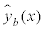(b=1,2,…,B) for each point of x=1,2,…,12. Comparing with the values derived from the true model (4), Tables 5 and 6 show the bias Bias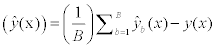, the mean squared error (MSE)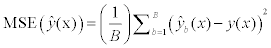, and the coverage probability CP(x) for a 95% confidence interval of B=1,000 sets for each x=1,2,…,12 based on the fitted curves produced by each procedure using the summarized data for true curves I and II, respectively. The means and standard deviations of estimated coefficients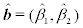are also shown as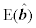and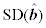in the tables. Note that the accuracy of the fitted curves cannot be measured based only on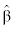, becauseand the accuracy must be affected directly by the positions of the knots. Figure 4 shows the curves joining the mean values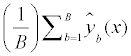Table 5: Comparisons of the estimated values for logRR0(x) on x=1,2,…,12, which were derived from the curves using procedure (i-iv) for Curve I, y=-0.2x–0.05x* with knots (3, 5, 7).

Table 6: Comparisons of the estimated values for logRR0(x) on x=1,2,…,12, which were derived from the curves using procedure (i-iv) for Curve II, y=-0.2x–0.05x* with knots (2.3, 3.2, 10.5).

Figure 4: Comparisons of curves joining the mean values of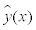with x=1,2,…,12 for true curves I and II.

First, procedure (iv), which used the likelihood-based assignment and the true knots, had a small bias and small MSE values in each scenario. It also gave closer coverage probabilities to 0.95, and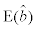was close to true b. Overall the curve produced using this procedure had a good fit to the true curve.

Next, the proposed procedure (i), which used the likelihood-based assignment and the selected knots, also had a small bias, small MSE values for each x. In particular, when x ≥ 9 for true curve I, it was shown that the curve produced using this procedure had a better fit to the true curve than that produced using procedure (iv). When x<5 for true curve II, this procedure had a slightly higher bias than procedure (iv). However, the coverage probabilities remained higher than 90%.

Procedure (ii), which used the midpoints assignment, was very similar to procedure (i) when x<5 for curves I and II. When x>5, the bias and MSE increased gradually, so this procedure could not deliver stable estimates with a large x.

Procedure (iii), which used the midpoints assignment and d0=0, estimated the value slightly higher with a low x compared with the other procedures. With a high x, it showed similar behavior to procedure (ii).

#### Discussion

In this paper, we proposed a procedure for assessing the nonlinear association between exposure levels and the risk of disease by assigning exposure levels to grouped exposure intervals. In particular, we focused on a restricted cubic spline model for J-shaped dose-response curves. The procedure can be applied to the log relative risks when they are given relative to the reference point x=0 and also to the interval x∈I0 . Our simulation results showed that the estimated curve was sensitive to the assignment, and the likelihood-based assignment could estimate the nonlinear association accurately. It showed that the proposed procedure using the likelihood-based assignment had a lower bias when used for estimation compared with other procedures that used the midpoints assignment.

One of the applications to estimate a dose-response association from summarized data is that it should be applicable to a metaanalysis. In general, the exposure categories were different in the studies so they should not be combined. Furthermore, in some of the meta-analysis studies described in Table 2, the reference category was also different, so it was inappropriate to combine them directly. Some methods have been discussed for meta-analysis to obtain the pooled estimate without estimating the association within individual studies. For example, Greenland and Longnecker  described the pool-first method for meta-analysis of trend involving data pooling before trend analysis. On the other hand, Rota et al.  proposed a random-effects meta-regression model for nonlinear dose-response relationship fitting second-order fractional polynomial models, where the twostep procedures requires initially fitting second-order fractional polynomial models within each study, and then pooling the studyspecific two trend components. They tried to fit a pool-first method on the data of a small number of studies, and they obtained the identical results achieved by using their random-effects approach. It may also be possible to estimate pooled curves using our proposed procedure by a multivariate random effect meta-analysis , as discussed in Larsson and Orsini . However, it has to be noted that both pool-first method and two-step procedure use pre-assigned exposure levels for grouped exposure intervals, and they assigned values by the midpoints assignment. Thus, the likelihood-based assignment could give different results in the pooled estimates. Moreover, in situations such as those for assessment of publication or other availability bias by the funnel plot, it is important to accurately estimate individually.

In the procedure reported herein, we fixed three knots in the J-shaped curve. The choice of the location of the knots is a crucial problem and the estimate of the curve was sensitive to their positions. Although we showed simulation results only for a situation of m=3 here, we also examined a procedure where the choice was based on likelihood for m>3, and the results of simulation studies could show that it produced well-fitted curves. In a similar manner to the procedure proposed herein, we can apply restricted cubic spline regression using other numbers of fixed knots to produce a more flexible curve shape. However, the choice of the number of knots is generally a crucial problem in spline regression. In this situation, the model with the best fit can be selected using a similar procedure by evaluating Akaike's Information Criterion (AIC), which is a penalized likelihood that takes into account the number of parameters estimated in the model based on likelihood (3). Also non-cubic spline models have been discussed in epidemiological studies. Further discussions of such models, including evaluations of different methods, are required in the future.

Our work, moreover, could be located in the errors-in-variable field, which aims to correct for bias that arises if measurement error in x is ignored. The statistical approaches developed in those fields might be applied in the situation discussed in this paper. We would also like to leave such a study including comparisons with the proposed procedure here, in our future work.

#### References

Select your language of interest to view the total content in your interested language

### Article Usage

• Total views: 12991
• [From(publication date):
December-2013 - Jul 17, 2019]
• Breakdown by view type
• HTML page views : 9140Can't read the image? click here to refresh